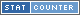# Welcome to Math NumbersTo the Greeks the word mathematics meant "knowledge and learning". The term mathematics has been shortened to math and refers to the science of numbers and their operations.

Math is the most used scientific principles used around the world by everyone, from a child counting fingers to engineers designing space ships. Mathematic operations are represented as equations, formulas or expressions.

Equation: An equation is includes an (=) equal signs between a left and right side. Both sides do not have to be a true answer to the each other, but if they are true it is also known as an identity.
Example: 5+x=7

Expression: Is like an equation but has no (=) equal sign so matching right side or solution.
Example: 2x6

Formula: A special type of equation is the formula, which shows the relationship between two or more variables.
Example: v = xyz when x = width, y = height, z = depth

### Searching For Math ProblemsThe easiest way to search this website for math equations is to use the search bar at the top of the page.

When using the search bar to find math equations and solutions simply type the expression in as so: "2+2" then press GO. Your search will forward you to the correct page.

You can also enter your math problem directly into the URL (or browser address bar) following the address "http://math-numbers.com/solve/" an example of this would be "http://math-numbers.com/solve/2+2". The url will accept more advanced entries as well such as "http://www.math-numbers.com/solve/(5+7.3(2))^-2"

### The Internet as a Math ToolThe Internet is comprised of millions of comptuers connected to computer, the computer is at the core an arithmetic logic apparatus able to perform millions to billions of mathmatical calculations per second.

It makes perfect sense to use the internet as another math tool, just as the slide rule then the calculator each new innevation offers greater flexibility, use and results.

Tools such as the calculator and this website are not meant to negate the need for people to understand how to perform math on their own.Addition also called adding uses the "+" plus symbol. When you add you combine two or more numbers together to get a total called a sum.

### Subtraction

Subtract

Subtaction uses the "-" minus symbol. When subtracting you remove a number from another nuber.

Examples for subtraction.

### Multiplication

Multiply

Multiplication also refered to as times is denoted with either the "x" symbol or "*" (typically when written) or astric symbol "*" astric symbol when used in computer languages or code.

When multiplying it is the first number and add it together as many times as their is the following number.

Examples for multiplication.

### Division

Divide

Division also known as dividing has many different ways it can be represented, "÷" symbol and the "/" or "\" are most common. Division is determining how many of one another can fit inside another number.

Examples for division.